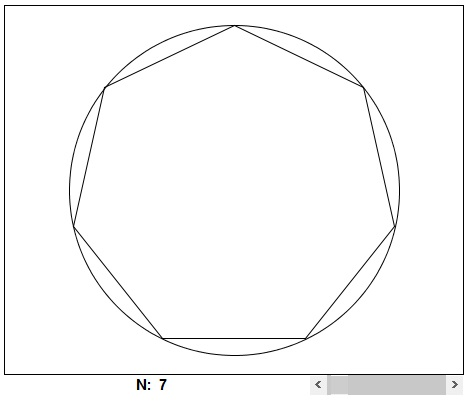# Estimating Circumference of a Circle

The applet below illustrates the manner in which the perimeters of inscribed regular polygons give an estimate to the circumference of a circle.

### If you are reading this, your browser is not set to run Java applets. Try IE11 or Safari and declare the site https://www.cut-the-knot.org as trusted in the Java setup.What if applet does not run?

If C is the circumference of the circle, pn an inscribed regular n-gon with perimeter Pn, is that true that

 limn→∞Pn = C.

As the number of sides n of the inscribed polygons grows without bound (i.e., "approaches ∞"), the perimeter (as a function of n) approaches a certain fixed value, which is naturally thought of as the perimeter (circumference) of the circle.

Why is that so? The fact has been established yet by Archimedes more than two thousand years ago. The perimeters of the inscribed n-gons form an increasing sequence. Archimedes also drew the circumscribed n-gons and showed that the perimeters of those form a decreasing sequence. Importantly, he also proved that the difference of the perimeters of the circumscribed and inscribed n-gons becomes increasingly smaller as n grows. (He gave estimates for n = 3, 6, 12, 24, 48, 96.) Archimedes did not possess a formal definition of limit, nor even a notion of real numbers. However, his approach can be easily formalized in a modern Calculus framework.

### References

1. S. Stein, Archimedes: What Did He Do Besides Cry Eurika?, MAA, 1999

### π: Applications and Calculations• Cavalieri's Principle
• Derivative of Sine and Cosine
• Distance From a Point to a Straight Line
• Maximum Volume of a Cut Off Box
• Mistrust Intuition of the Infinite
• Naturally Discontinuous Functions
• Rolle's and The Mean Value Theorems
• Function, Derivative and Integral
• Area of a Circle by Rabbi Abraham bar Hiyya Hanasi
• Schwarz Lantern
• Two Circles and a Limit
• Deceptive Appearances
• Problem 4010 from Crux Mathematicorum
•